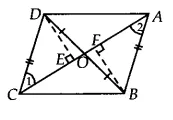Q

# In Fig. 9.25, diagonals AC and BD of quadrilateral ABCD intersect at O such that OB = OD. If AB = CD, then show that: (iii) DA || CB or ABCD is a parallelogram.

Q : 6     In Fig. , diagonals AC and BD of quadrilateral ABCD intersect at O such that . If , then show that:

(iii) or ABCD is a parallelogram.

[Hint : From D and B, draw perpendiculars to AC.]ViewsSince DCB and ACB both lie on the same base BC and having equal areas.
Therefore, They lie between the same parallels BC and AD